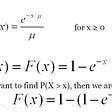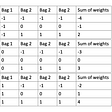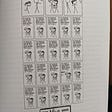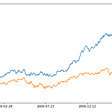# Volatility Smile and Delta Hedging (Part 2) — Down the rabbit hole of Smile Risk Hedging.

So we continue our journey down the rabbit hole of volatility surface and vega risk exactly where we left off last time (if you want to refresh your memory, hop back to Part I ). As we recall, our theoretical options book took a massive hit after the sudden drop in S&P500 and spike in volatility. Our vega loss is approximately \$480K, and our head of risk team is not having the best day…

As we assess our position, risk, and different scenarios, we can clearly see that our biggest risk at the moment comes from the vega. Our current Gamma exposure is less significant for two reasons:

1. There is a negative relationship between Gamma and Time — the longer our option, the less sensitive the option to the move of the underlying asset.
2. There is a negative relationship between Gamma and Volatility — Higher volatility level reduces the option's gamma (if you are interested in exploring the relation between Vol-Gamma-Theta, take a look at this blog ). Furthermore, because of the volatility smile shape (i.e., premium for puts over calls in vol term), we were originally slightly long Gamma, which means that as the spot moved down, we were actually in positive gamma territory.

The plots below emphasize the effect time and moneyness have on the options book Gamma. When we take a snapshot of our Gamma exposure off spot/vol matrix, we see it’s pretty flat, which means that our Gamma exposure is somewhat negligible.

So let’s focus on our vega risk for a moment. Our portfolio has currently about negative \$80K vega exposure, meaning that any 1 vol-point move will have an ~\$80K on our P&L (so if volatility goes down, 1 vol will incur ~80K profit, and if volatility moves up, we will incur a loss of about the same amount).

As our risk department is quite strict when it comes to P&L variance swings and risk limits, we are told to reduce our net vega exposure to zero (something to do with the fact that a large PM at our firm got hit massively on long-dated bonds and the head of risk is terrified..). Obviously, the most intuitive and straightforward way to offset our vega risk is to buy options, as this will add long vega exposure to our book and reduce the sensitivity of our book to a parallel shift in volatility. However, there are two major caveats to do that:

1. Frequently hedging vega risk using options is costly (high transaction cost and fees)
2. Any option that we add to our book adds another subset of exposures — Think about an option as a subset/vector of risks (say, an array of MTM+ Greeks). By adding options to our book, we accumulate multiple subsets, which can turn our options book risk profile into something we might not intend to (in addition to multiple pin-risks for specific strikes in our options book)

So we established that frequently trading options as a vega hedge is inefficient, so how can we efficiently hedge that risk? Well, we can always turn to our good old underlying asset (trading spot/forward/future) and use it to hedge our vega. Now, I know what most of you think right now: “how the hell can a linear product be used to hedge a non-linear risk (with a convex nature) like vega?” This is where options theory and academic literature start to diverge from the practice…

# Breaking down Smile Risk

Before discussing Delta Hedging IRL trading (which you will soon see vary significantly from the Delta we all know as B&S Delta), we need to talk about Smile Risk.

So what is the Smile Risk of our options book? Well, as implied by the name, it is the risk that arises from the volatility smile shape and corresponds to vega-related exposures (i.e., Vega/Vanna/Volga). As they can all be denominated in absolute terms (say \$), we can aggregate them into a neat function.

Now, if this seems too intimidating, don’t worry; we will shortly see that this is rather intuitive…

So our options book risk subsets can be obviously aggregated, so if we focus solely on our vega-related risks, we can sum them as shown above. If we want to estimate our Vanna effect on our vega risk, we need to multiply it by the change in spot. In contrast, if we want to estimate our Volga effect on our vega risk, we need to multiply it by the vol change (but because each option possibly has a different vol mapped to it, we need to use the corresponded vol). After aggregating all vega-related risks, we need to estimate the move in vol as a function of change of spot (which we will later see is really model-dependent). If we plot our aggregated smile risk (without accounting for ∂Vol∂Spot)

We are now one step closer to understand how all the things we have seen so far come together and help us as practitioners with the most active part of our options book risk management — Delta Hedging. Let’s take a deep breath before we dive deep into the essence of volatility trading.

# Delta Hedging and Strike Conventions

Before we begin, I want to make it clear — Delta is like opinions; every trader got one (I saved you the explicit quote…). Although we were taught/told/read online that the delta calculation returns a unique value given a set of parameters (which is true IF we are talking about B-S “theoretical” delta), IRL trading delta calculation is highly model (and parameters/assumption) dependent.

Let’s start with acknowledging that the B-S model assumes continuous delta hedging (which is practically impossible IRL), but the matter of fact is that we hedge discretely. Furthermore, we already know that the B-S delta underestimates changes in volatility level and cross effects between spot and volatility (and we’ve already seen that changes in volatility affect Gamma and Delta). Lastly, as we’ve seen earlier, moves in volatility, in most cases, are correlated to spot moves, so it only makes sense to incorporate that effect into our delta calculation and hedge our vega exposure with an adjustment to the “theoretical” delta. Generally speaking, we can rewrite our delta to account for the smile risk as follows:

That kind of makes sense, right? We add the vega (and vega-related exposures) to our delta and multiply it by the expected change is vol as a function of spot change. But how can we quantify how much vol is LIKELY to move when the spot moves? To estimate the likely move in vol, we need to take a trip down memory lane to our high-school geometry lessons…

If we think about it, the volatility smile is a representation of vol (on the y-axis) corresponded with strikes (on the x-axis), so, theoretically speaking, we can convert “strikes” into “likely spot scenario” and calculate the slope of the curve :

Or if you want a graphic representation to support this calculation:

Another feature of this plot that we can observe (although not so noticeable) is that the slope itself is not constant (i.e., the volatility skew is not linear), this means that the rate of the change in slope is positive (or in other words- as volatility increases, the rate of the volatility change increases, which means that volatility has a convexity, or in other words vol-of-vol >0).

Cool right? So we know that as the spot moves X points lower, the vol will increase by Y vol points higher, which gives us a decent estimation of how much our smile risk will affect our options book MTM (and P&L as a byproduct) and also allows us to account for that change and offset it with our delta.

Now, let’s go back to our RL example of a short 3-month RR (4130/4610) that we happened to sell one day before the market crashed and burned… Our initial delta was 50delta (we were long 25delta call + short 25delta put). Converting this delta to a \$term, it was approximately \$26mio (or about 110 ES futures); as we move left on the spot/strike axis, our delta increases. The below chart emphasis the difference between the B-S delta and smile-adjusted delta.

As we can see, our smile-adjusted delta is marginally higher than the “theoretical” delta, which means that we need to sell more (or hold less) delta at any given point to hedge our vega (and vega-related) exposures. That actually makes sense, right? if we know that volatility increases as the spot moves lower, we can hedge that negative effect on our P&L by selling more (or holding less) units of the underlying asset. Still, like everything in financial markets, this is far from being as easy as I portraited, and we will soon understand why…

## Sticky Strike or Sticky Delta — What is your flavor of choice?

Anyone who has ever traded in different markets can tell you that not all markets were created equal. Trading in the Equity market differs significantly from trading in the Rates market (or FX), and trading in the Commodities market differs significantly from trading in the Credit market. That means that different practices are applied when it comes to quoting conventions in the volatility space. There are two quoting conventions used by options traders: Sticky Delta and Sticky Strike.

## Sticky Delta/Moneyness

The Sticky Delta convention is mostly used by FX option traders in the OTC market. This quoting convention means that the implied volatility level of any option is dependent on a FIXED DELTA strike (for example, 25-delta put/call)

As we can see above, the Sticky Delta convention means that as the spot moves lower, the “fixed-delta” strikes remain “sticky” (or unchanged)

## Sticky Strike

The Sticky Strike Rule means that the volatility for each strike is independent of a movement in the underlying asset (aka, “fixed-strike volatility”).

As we can see above, as the spot moves lower, the level of implied volatility for fixed strikes (4300 in our example) remains unchanged relative to where it was when the spot was at 4400.

Now, you are probably wondering why I spent the time to explain the difference between the two quoting conventions. The reason for that is that each quoting convention affects our calculation of the smile adjustment we apply to the B-S delta (or dVol/dSpot to be exact). If we were to use the Sticky Delta rule, for a small move lower in the spot, the implied volatility for a fixed strike actually declines (as the entire smile shifts vertically). If we use the above chart, when we moved from 4400 to 4300, the volatility of the 4300 declined from ~15.3 to 15, whereas if we were to use the Sticky Strike rule, the volatility (again, for a small move lower in the spot) would have been unchanged. In volatility trading jargon, that’s called “riding the smile” (be sure to look for Emanuel Derman’s paper about that in my shared drive).

“Riding the smile” basically means that we take advantage of the quoting conventions (or arbitraging) to profit from a move in implied volatilities resulted from a move in the underlying spot. In a Sticky Strike world, we would incur no profit/loss as spot moves (as the volatility of a fixed strike is independent of the spot dynamic). In both cases, smile-delta hedging a short-vega position should actually outperform the B-S delta in a declining market while underperforming the B-S delta in a rallying market. WAIT!, WHAT?!

I sense that most of you by now are dazed and confused. We’ve been spending the better part of a two-part blog to talk about volatility smile, smile risk, and how to incorporate that into our delta hedging just to end up saying that when we hedge with smile delta, we are exposed to the direction of the market (the exact opposite of what delta hedging should do!). Fear not; I will make everything clear by the end of this blog…

Even though the quoting conventions both for a sticky strike and a sticky delta/moneyness imply an arbitrage, IRL trading this arbitrage, in some cases, is not feasible, and why is that? If you read closely, you see that riding the smile applies for relatively small moves (in spot/time-space). You can be sure that a sudden drop of spot (say 2–3% out of the blue) would send market participants buying vol and eventually shift the entire volatility smile higher (in other words, the realized move in implied volatility will outperform the implied skew/vol-convexity). On the other hand, a move higher in spot (while not a sharp short-term move) should weigh on the implied volatility level and eventually cause a parallel shift lower of the entire volatility smile (even if we ignore 2nd order effects like lower RR and BF).

If we take S&P500, for example, we can observe a clear negative correlation between the underlying spot and VIX (which is a good gauge for the 1-month implied variance). However, if we run the same test for USDJPY, we get a worse fit (lower R², with several observations extremely far from the regression line).

So what is the “right” approach when it comes to estimating the spot-vol relation? should we assume sticky strike or sticky moneyness? well, the answer lies somewhere in the middle… As volatility is more of a clustering issue (or regime clustering to be exact) than a prediction of value, it really depends on the market regime. When the market is nervous (in a risk-off, for example) implied volatility tends to overperform the smile, so at minimum, we should assume that strikes are sticky, but when the market is drifting, more often than not implied volatility tends to underperform the smile (so assuming sticky moneyness/delta is more adequate in that case.). As I said earlier, our smile-adjusted delta is highly sensitive to our model/assumption.

# Few words about volatility surface modeling…

While this entire blog post was about understanding volatility surface properties, smile risk, and managing smile risk, we never really touched the core of implied volatility, and that’s the modeling of implied volatility surface. The main reason is that modeling implied volatility smile is a heavily mathematical subject, which doesn’t fit the scope of this blog (or at least this specific blog post), but I want to touch on one point that’s worth mentioning which is the sensitivity of our smile risk management to the choice of volatility model and parameter calibration.

First, different markets have different price dynamics, which means that there is not one volatility model that fits all. If we look at the Rates market dynamic, for example, one would probably choose the SABR model that has the capability of handling negative strikes, while in FX most practitioners tend to use a variation of the Vanna-Volga model. In any case, even if we choose the appropriate model, we still (in most cases) need to calibrate our controlling parameters (spot/vol correlation and vol-of-vol for example, in the Heston model), which are not directly observed in the market. That makes our assessment of volatility dynamic highly sensitive to how accurate we calibrate our parameters to the actual dynamic. The below chart shows two different models (Heston and Vanna-Volga) under the same ATM price and implied spot-vol correlation/vol-of-vol. As we can see they don’t fit perfectly, even after we try to minimize the square errors…

So this warps our journey throw volatility smile and delta hedging… I really hope this subject turned less intimidating for novice practitioners and provided some insight for more veteran vol gals/guys. Obviously, there is much more to discuss, as we barely scratched the surface (pun intended).

--

--

--

## More from Harel Jacobson

Love podcasts or audiobooks? Learn on the go with our new app.

## Statistics: Maximum likelihood estimation## Distributions## Continuous random variable distributions | Probability theory | Part 7## 5+ Reasons I Fell in Love With Math — II## Determine Weight of Counterfeit coins [Asked in GS Interview]## How not to be wrong — The hidden maths of everyday life by Jordan Ellenberg## How to delta hedge an option: Part II## Buy on gap strategy and its performance over time## The Elastic Volume Weighted ATR : Breakouts Highlighting Using Volume and Volatility## Think Like a Market Maker — Understanding Implied Volatility